# NATA Mock Test - 1

## 50 Questions MCQ Test NATA - Sample Paper & Mock Tests | NATA Mock Test - 1

Description
Attempt NATA Mock Test - 1 | 50 questions in 90 minutes | Mock test for JEE preparation | Free important questions MCQ to study NATA - Sample Paper & Mock Tests for JEE Exam | Download free PDF with solutions
QUESTION: 1

### Following graph is plotted between displacement and time. Its acceleration is best described as (where b, c are +ve constants)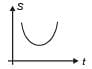Solution: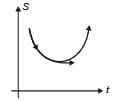From the graph it is clear

Velocity is the slope of displacement-time graph. It is first negative, then zero and finally, positive. Hence, velocity continuously increasing so acceleration must be always positive.

QUESTION: 2

### For acute angle θ, find value of sec2θ−tan2θ

Solution: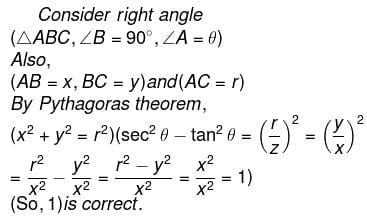QUESTION: 3

### The oxidation states of S-atoms in Marshall’s acid and Caro’s acids are respectively

Solution:

Marshall’s acid is H2S2O8 and Caro’s acid is H2SO5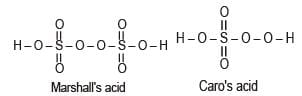O state of S = +6

QUESTION: 4

First ionisation energy is lowest for

Solution: Sn has minimum first ionisation enthalpy.

QUESTION: 5

An electron return from excited state to ground state in H-atom and emits a photon of wavelength l, the principle quantum number of excited state is given by

Solution: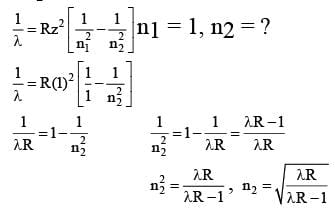QUESTION: 6

If resistance R = (100 ± 5) Ω and current I = (10 ± 0.2) A. The percentage error in thermal power (P = I2R) is

Solution:

As P = I2R

Hence, relative error in thermal power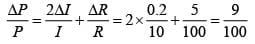Hence, % error in power = 9%.

QUESTION: 7

If the system of linear equations : x+ 3y +7z = 0 -x + 4y +7z = 0 (sin3θ)x+ (cos2 θ)y +2z = 0 has a non-trivial solution, then the number of values of θ lying in the interval [0, π], is

Solution: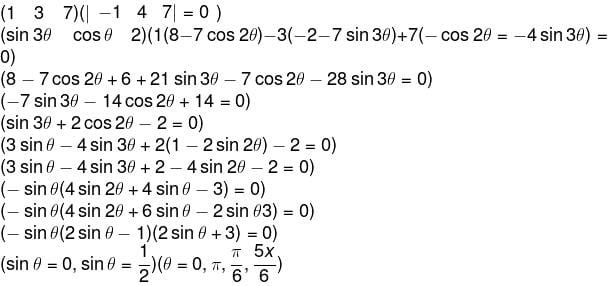QUESTION: 8

The number of integral values of m for which the equation, (1 + m2) x2 – 2(1 + 3m) x + (1 + 8m) = 0, has no real root, is:

Solution: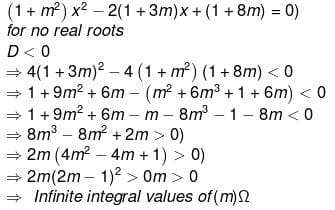QUESTION: 9

The negation of A →(A v ∼ B) is

Solution: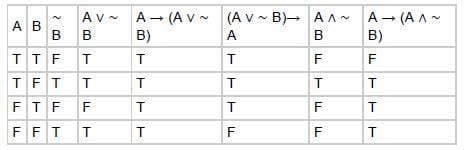QUESTION: 10

Which of the following compound does not decompose on heating?

Solution:

Na2CO3 does not decompose whereas NaHCO3, MgCO3 and Ca(HCO3)2 decompose on heating

QUESTION: 11

If a particle moving in a straight line with uniform acceleration starting from rest covers displacement S1 in pth second and displacement S2 in (p + 1)th second, then the displacement upto p seconds is given by

Solution:

Displacement in nth second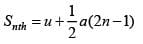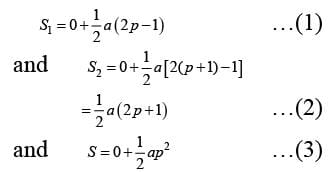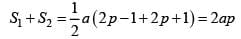Substituting the value of a from equation (3)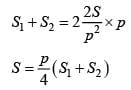QUESTION: 12

If f is a function of real variable x satisfying f(x + 4) – f(x + 2) +f(x) = 0, then f is a periodic function with period:

Solution:

f(x + 4) – f(x + 2) + f(x) = 0 f(x + 6) – f(x + 4) + f(x + 2) = 0

∴ f(x + 6) + f(x) = 0

⇒ f(x + 12) + f(x + 6) = 0

∴ f(x + 12) = f(x)

⇒ f(x) is periodic with period 12.

QUESTION: 13

A box contains 5 black and 4 white balls. A ball is drawn at random and its colour is noted. The ball is then put back in the box along with two additional balls of its opposite colour. If a ball is drawn again from the box, then the probability that the ball drawn now is black, is

Solution: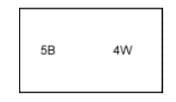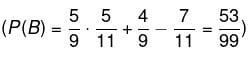QUESTION: 14

A particle is moving in uniform circular motion with speed v of radius R. The ratio of average velocity to average acceleration in half of the circle

Solution: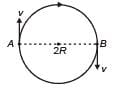Average velocity from A to B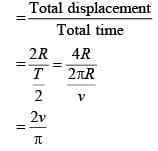Average acceleration from A to B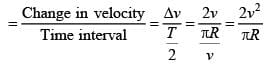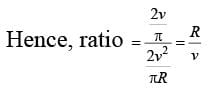QUESTION: 15

Lest S = (z ε C : z(iz1 - 1) = z1 + 1, |z1| + 1, Then, for all z ε S, which of the following is always true?

Solution: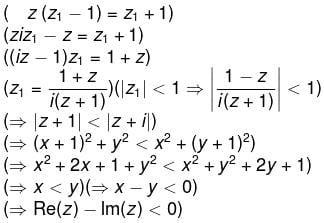QUESTION: 16

Find the odd one out. 8, 27, 64, 100, 125, 216, 343

Solution: he pattern is 23, 33, 43, 53, 63, 73. But, 100 is not a perfect cube.

QUESTION: 17

The drawing represents a flat piece of sheet. The dotted lines show where the sheet has to be folded. Find the object that can be made by folding the sheet.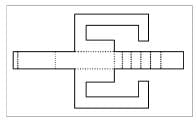Solution: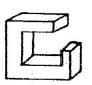QUESTION: 18

Find the mirror image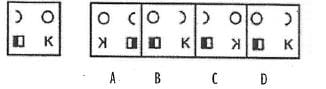Solution: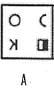QUESTION: 19

Which one of the answer figures, shows the correct view of the 3-D problem figure, after the problem figure opened up.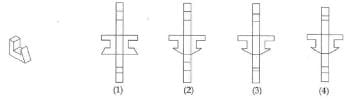Solution: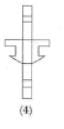QUESTION: 20

Bibi ka maqbara’ bears a striking resemblance to which famous monument?

Solution: The Bibi ka Maqbara is a tomb located in Aurangabad, Maharashtra, India. It was built by Mughal emperor Aurangzeb's son Azam Shah in the memory of his mother. It bears a striking resemblance to the famous Taj Mahal, the mausoleum of wife of Shah Jahan. He had built the Badshahi Mosque at Lahore one of the largest Mosques in the world and the largest one at that time, as well as the small, but elegant, Pearl Mosque at Delhi.

QUESTION: 21

_____________is generally used as reinforcement in RCC.

Solution: Reinforced Cement Concrete (R.C.C.) is the combination of ordinary concrete with the reinforcement to increase its compressive and tensile strength to a great extent. Concrete is a versatile material for modern construction which is prepared by mixing well proportioned quantities of cement, sand, crushed rock orgravel and water.

It has been used from foundations to the rooftop of the buildings, in the construction of highways, roads traffic etc.

QUESTION: 22

Find the odd one out. 396, 462, 572, 427, 671, 264

Solution: Each of the numbers except 28, is a multiple of 3.

QUESTION: 23

Select from the alternatives, the cube that can be formed by folding the following sheet: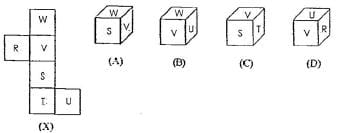Solution: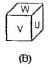QUESTION: 24

What is the value of angle of response for clay(dry) soil.

Solution:

The depth of footing should also be such that the rate of angle of spread of the load from the wall base to the outer edge of ground bearing does not exceed the permissible value.

QUESTION: 25

_______________is the world’s 2nd largest stadium and is located in India

Solution: 1st Largest Stadium- Budh International Circuit with 1,20,000 capacity (Greater Noida), UP. 2nd Largest Stadium- Salt Lake Stadium, Kolkata with 80,000 capacity(Kolkata) 3rd Largest Stadium- Jawahar Lal Nehru Stadium, New Delhi

QUESTION: 26

Which of the answer figures is the correct water reflection of the problem figure?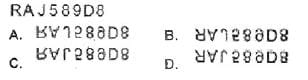Solution: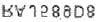QUESTION: 27

Insert the missing number. 16, 33, 65, 131, 261, (....)

Solution: Each number is twice the preceding one with 1 added or subtracted alternatively.

So, the next number is (2 x 261 + 1) = 523.

QUESTION: 28

Out of the given figures, four are similar in a certain way,one is not. Which figure does not belong to the group?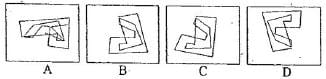Solution: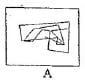QUESTION: 29

______________is the architect of ‘Bharat Bhavan’ in Bhopal.

Solution: Charles Correa widely known for his works in contemporary architecture, played an important and vital role in the development of architecture in India after Independence. His principles of designing includes prevailing resources, energy and climatic aspects to create and determine order of space. His works include the Chapalimaud Foudation Centre in Lisbon, also famous by the name "Project to the Unknown", the Sabarmati Ashram in Ahmedabad and the Madhya Pradesh Legislative Assembly in Bhopal. Craft Museum, New Delhi, Bharat Bhavan, Bhopal, Jawahar Kala Kendra in Jaipur are some other examples of his intellectual knowledge.

QUESTION: 30

Identify the building in the given image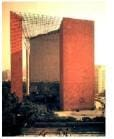Solution: LIC of India Head Office Address: LIC of India Company Limited Registered Office, Jeevan Bima Marg, Nariman Point, Mumbai, Maharashtra

QUESTION: 31

The indentation on the top of a brick is called

Solution: The depression provided in the face of brick during its manufacturing is known as frog of bricks. Depth of the brick is 10mm to 20mm. If the bricks are laid according to British standards, the frog should be laid upward and filled with mortar. When the frog is laid upwards, the load is evenly spread throughout the width of the brick all the way down to the foundations. If the frog is laid down, the load is forced to the outsides of the brick.

QUESTION: 32

Which of the following buildings is NOT designed by Hafeez Contractor?

Solution: Hafeez Contractor is an Indian architect. He has designed many skyscrapers in India, primarily in the city of Mumbai.

QUESTION: 33

Find the odd one out. 3, 5, 11, 14, 17, 21

Solution: Each of the numbers except 14 is an odd number.

The number '14' is the only EVEN number.

QUESTION: 34

Which one of the following is not built in red sandstone?

Solution: Golkonda, also known as Golconda, is a citadel and fort in Southern India and was the capital of the medieval sultanate of the Qutb Shahi dynasty (c.1512–1687), is situated 11 km (6.8 mi) west of Hyderabad. Golkonda Fort was first built by the Kakatiyas as part of their western defenses along the lines of the Kondapalli Fort. The city and the fortress were built on a granite hill.

QUESTION: 35

___________is the ancient science of construction / architecture.

Solution: Vastu shastra is a traditional Hindu system of architecture which literally translates to "science of architecture." These are texts found on the Indian subcontinent that describe principles of design, layout, measurements, ground preparation, space arrangement, and spatial geometry.Vastu Shastras incorporate traditional Hindu and in some cases Buddhist beliefs.

QUESTION: 36

The problem figure in the box on the left has one identical figure from amongst A, B, C and D on the right. Find the figure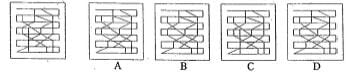(A) a

(B) b

(C) c

(D) d

Solution: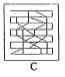QUESTION: 37

The famous Empire State building is in which of the following cities?

Solution: The Empire State Building is a skyscraper in New York City, United States. It is 381 meters (1,250 feet) tall and has 102 floors, and the height to its pinnacle is 1,454 feet.

QUESTION: 38

If a sheet of paper is folded two/three times from the centre and cuts are made as shown, how will it appear when it is opened?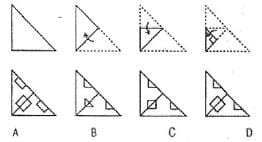Solution: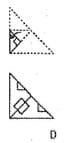QUESTION: 39

The drawing represents a flat piece of sheet. The dotted lines show where the sheet has to be folded. Find the object that can be made by folding the sheet.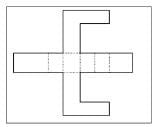Solution: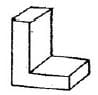QUESTION: 40

Arrange the objects given the question figure, according to the stated manner and choose the correct composite figure.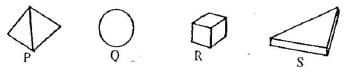S between P & R, R on the ground, Q touching S only

Solution: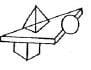QUESTION: 41

The problem figure in the box on the left has one identical figure from amongst A, B, C and D on the right. Find the figure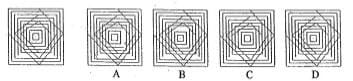Solution: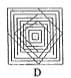QUESTION: 42

Insert the missing number. 10, 5, 13, 10, 16, 20, 19, (....)

Solution: There are two series (10, 13, 16, 19) and (5, 10, 20, 40), one increasing by 3 and the other multiplied by 2.
QUESTION: 43

Identify the building from the given image.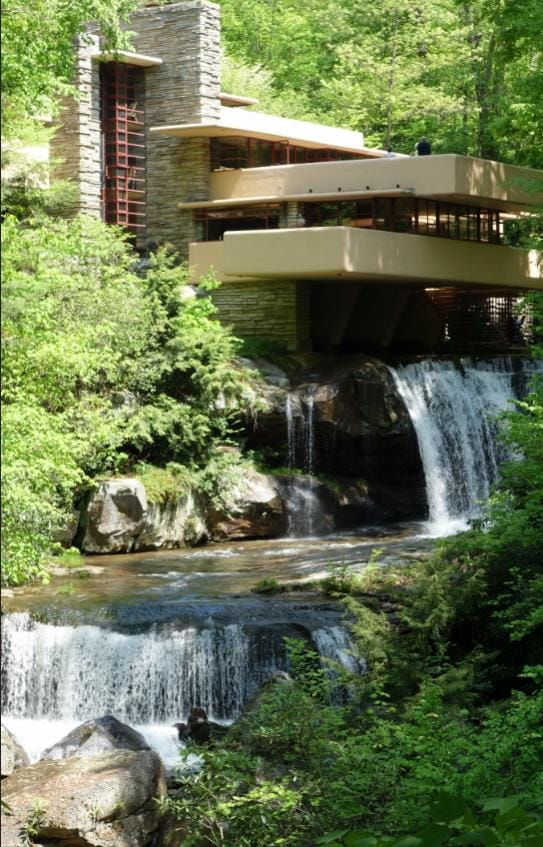Solution:

Fallingwater is a house designed by architect Frank Lloyd Wright in 1935 in rural southwestern Pennsylvania, 43 miles southeast of Pittsburgh

QUESTION: 44

Out of the given figures, four are similar in a certain way,one is not. Which figure does not belong to the group?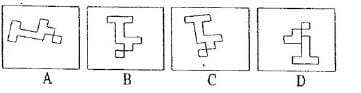Solution: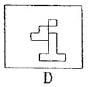QUESTION: 45

Every great architect necessarily - a great poet. He must be an original interpreter of his time, his day, his age. Was quoted by which famous architect ?

Solution: Frank Lloyd Wright (June 8, 1867 – April 9, 1959) was an American architect, interior designer, writer, and educator, who designed more than 1,000 structures, 532 of which were completed. Wright believed in designing structures that were in harmony with humanity and its environment, a philosophy he called organic architecture. This philosophy was best exemplified by Fallingwater (1935), which has been called "the best alltime work of American architecture".

QUESTION: 46

Out of the given figures, four are similar in a certain way,one is not. Which figure does not belong to the group?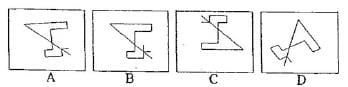Solution: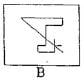QUESTION: 47

Arrange the objects given the question figure, according to the stated manner and choose the correct composite figure.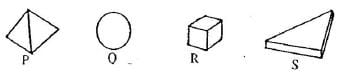R on the ground only, P touching S only, P between Q & R

Solution: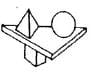QUESTION: 48

Find the odd one out. 10, 25, 45, 54, 60, 75, 80

Solution: Each of the numbers except 54 is multiple of 5.

QUESTION: 49

Arrange the objects given the question figure, according to the stated manner and choose the correct composite figure.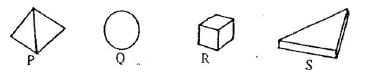Q on the top, P touching Q, R between S and P.

Solution: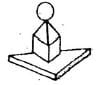QUESTION: 50

Find the odd one out. 6, 9, 15, 21, 24, 28, 30

Solution:

Each of the numbers except 28, is a multiple of 3Use Code STAYHOME200 and get INR 200 additional OFF Use Coupon Code